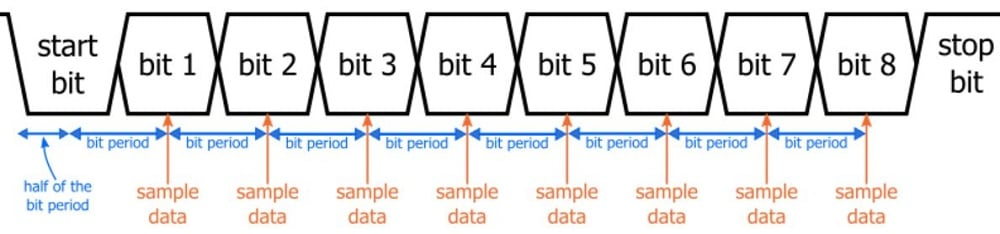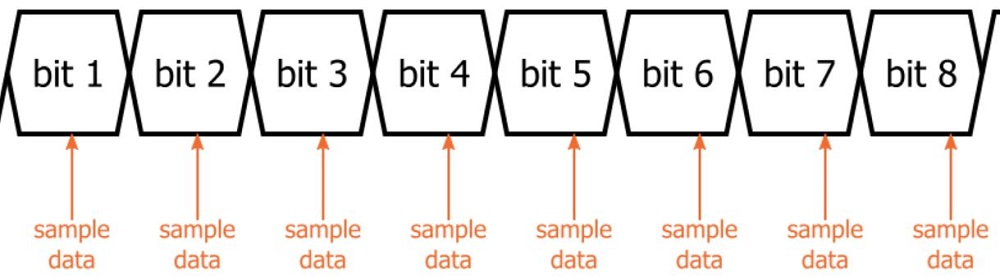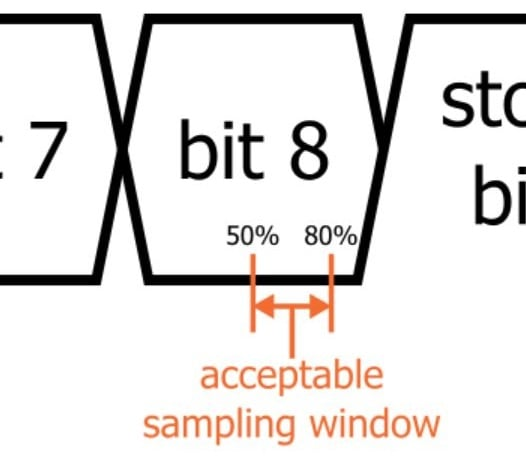Technical Article

# UART Baud Rate: How Accurate Does It Need to Be?

January 25, 2017 by Robert Keim

### Related Information

I really appreciate the convenience offered by microcontrollers that have a fairly-high-accuracy internal oscillator. But these oscillators are never as precise as a crystal, so there is always a lingering doubt—is the internal oscillator accurate enough for all the timing functionality that I might need?

One of the most common timing-sensitive tasks is UART communication. The interface lacks an external clock, and the Tx and Rx devices can reliably share data only when their internal baud rates are equal.

But of course “equal” is not really an engineering word—we always have to account for noise and error and variation, and in the case of a microcontroller’s internal oscillator, we’re not talking about a few parts per million. In my experience, microcontrollers rarely offer an internal oscillator that has accuracy better than ±1.5%. Two microcontrollers with ±1.5% clock sources will have baud rates that are different by as much as 3%. Is that good enough?

### Baud Rate, Bit Period, and Sampling

(Note: For the remaining analysis we’ll use the Tx device as the reference; in other words, we’ll assume that the Tx device is always at the nominal baud rate and the Rx device is at the inaccurate baud rate.)

The first thing to keep in mind is that the only relevant error is the difference between the transmitter’s baud rate and the receiver’s baud rate (as opposed to the difference between an expected baud rate and an actual baud rate). For example, you will have no difficulty transferring data if your two communicating devices are both operating at 9800 baud, even if the expected rate (based on the nominal clock frequency) is 9600 baud.

The following diagram shows the basic timing procedure involved in UART reception. If the receiver baud rate perfectly matches the transmitter baud rate and the first bit is sampled at the exact middle of the bit period, the last data bit will also be sampled at the exact middle of the bit period.If the baud rates (and thus the bit periods) are different, each sampling point will move gradually closer to the bit transition. In other words, the last data bit is the one that is most severely affected by baud rate discrepancy. Note: For convenience, we’ll always assume that the Rx bit period is longer than the Tx bit period; the result would be the same if we assumed a shorter Rx bit period.### Solving for Maximum Allowable Error: The Simpler Version

Based on this observation, we can first propose the following: the baud rates are sufficiently accurate if the last data bit is sampled before the transition from last data bit to stop bit. (We’re assuming here that the system can tolerate an improperly sampled stop bit.) But we don’t want to sample one nanosecond before the expected transition; we need some margin. I think a reasonable margin is 20%—i.e., the last bit must be sampled at least 20% of the bit period before the transition from last data bit to stop bit.The bit period for the Tx device is defined as follows:

$$T_{TX}=\frac{1}{baud rate}$$

If we use E to refer to the absolute value of the percentage difference between the baud rate of the receiver and the baud rate of the transmitter, we have the following for the additional bit-period time on the Rx side:

$$\Delta T_{RX}=T_{TX}\cdot\left(\frac{E}{100}\right)$$

For this first analysis, we’ll simplify by assuming that the timing procedure begins in the exact middle of the start bit. So for an eight-data-bit interface, the sampling time for the last data bit will be off by 8ΔTRX. Thus, our is-it-accurate-enough condition is the following:

$$8\Delta T_{RX}<0.30T_{TX}$$

because we said that the acceptable sampling window for the last data bit is from 50% (the ideal value) to 80% (as close to the transition as we are willing to go), and 80% – 50% = 30% = 0.3.

We can solve for maximum allowable E as follows:

$$8\Delta T_{RX}<0.30T_{TX}$$

$$\Rightarrow8\left(\frac{E}{100}T_{TX}\right)<0.30T_{TX}$$

$$\Rightarrow E<3.75$$

So, based on this simplified analysis, eight-data-bit UART communication should be reliable as long as the difference between transmitter baud rate and receiver baud rate is less than 3.75%.

### Maximum Allowable Error: The Complete Analysis

The previous section gives you a rule of thumb for any eight-data-bit UART system that can tolerate frame errors (i.e., errors indicating an improperly sampled stop bit). In this section, we’ll develop a comprehensive equation that can provide a more precise and customized estimate.

To do this, we will incorporate the following:

• variable location of start-bit sampling
• variable number of data bits
• variable margin for sampling the final bit
• presence or absence of a parity bit
• frame-error tolerance

From the previous section, we have

$$8\Delta T_{RX}<0.30T_{TX}$$

Now our final-bit-sampling margin is a variable; we’ll use M (previously we used 20%). As you can see from the following equation, M must be entered as a decimal, not a percentage.

$$8\Delta T_{RX}<\left((1-M)-0.5\right)T_{TX}$$

$$8\Delta T_{RX}<\left(0.5-M\right)T_{TX}$$

We still have the 8 out in front of ΔTRX. We need to change that because now the number of data bits (denoted by N) is a variable. We also will include a variable for the parity bit (P) and the stop bit (S). If you have a parity bit, use 1 for P; if not, use 0. If you want to ensure that the stop bit is sampled correctly, use 1 for S; if not, use 0.

$$(N+P+S)\Delta T_{RX}<\left(0.5-M\right)T_{TX}$$

The last thing we need is the variable that accounts for the actual position at which the start bit is sampled. The equation in its current form assumes 50% (i.e., the exact middle of the bit period). This is the ideal position. Any deviation from the ideal can cause the sampling position of later bits to be closer to the transition (and thus closer to an error). We will incorporate this into the equation by adding the following term to the left-hand side:

$$D_{SB}T_{RX}$$

This term refers to the deviation from the ideal start-bit sampling position, expressed as a decimal multiplied by the receiver’s bit period. So, for example, if the start bit is sampled 60% of the way through the bit period, this term would be |(50% – 60%)|TRX = 0.1TRX. You can’t readily determine an exact value for this term, but if you know the details of your UART’s low-level functionality, you could come up with a reasonable worst-case estimate.

Now our equation is

$$D_{SB}T_{RX} + (N+P+S)\Delta T_{RX}<\left(0.5-M\right)T_{TX}$$

If we recall that the Rx bit period is equal to the Tx bit period plus the additional time corresponding to the error percentage, our final equation is as follows:

$$D_{SB}\left(\left(1+\frac{E}{100}\right)T_{TX}\right) + (N+P+S) \left(\frac{E}{100}T_{TX}\right)<\left(0.5-M\right)T_{TX}$$

### Example and Conclusion

Let’s consider the following system:

• margin is 30%
• worst-case start-bit sampling deviation is 5%
• eight data bits
• no frame errors allowed
• parity bit is used

Thus, we have

$$0.05\left(\left(1+\frac{E}{100}\right)T_{TX}\right) + (8+1+1) \left(\frac{E}{100}T_{TX}\right)<\left(0.5-0.3\right)T_{TX}$$

$$\Rightarrow \ \ \left(0.05+\frac{0.05E}{100}\right) + \left(\frac{10E}{100}\right)<0.2$$

$$\Rightarrow \ \ E<1.49$$

I hope this article helps you with any future baud-rate-accuracy concerns, and remember that a careful baud-rate analysis may indicate that you can confidently omit an external crystal, even if your internal oscillator is not as precise as you might like.• F
fool February 01, 2017

While this is great in theory, how about some more details about praxis?
In other words: how tolerant are the real-world implementations that modern microcontrollers use, with hardware interrupts for start-bit detection, and 8x or 16x oversampling? Also, since internal oscillators are mainly temperature-intolerant, if both devices that are communicating are in the same environment (subject to the same temperature differences), it would actually probably be better if both were using an internal RC, or both using a crystal, rather than one the RC and one a crystal…

Like.
• S
simne February 03, 2017

To figure out needed accuracy of frequency generator, one should consider real implementation of UART with which will work.
Because different implementation will have different sensitivity to frequency shift.

I know at least three variants:
1. Naive implementation, something similar to considered in article (I have not seen examples).
2. Implementation with 4x samples per bit, and with digital PLL-like system, which constantly monitors edges of bits and choose sample position nearest to center of bit. It’s tolerated about 5% shift of frequency. For example Zilogs UARTs uses this.
3. Implementation with more than 3x samples per bit, and averaging form these samples (or choose middle bit, configurable). Cypress UARTs uses this.

Like.
•Fixup February 09, 2017

This is an interesting topic and I can add some experience.  I have put around 1000 systems into the field that use Atmel micro’s with their internal oscillator, which communicate with a display a few metres away via UART at 9600 baud. I have observed around 5% would fail outright because of baud rate mismatches, even with chips out of the same tubes. Around another 5% would experience ‘junk’ on the character display over time where temperature shifts have affected the devices.  To fix this I added a ‘trim’ feature to each micro where I adjust a an Atmel calibration byte to centre the frequency of each micro to a known value.  This value is then stored in non-volatile memory and used each time at startup.  Following this method for the past 2 years has brought baud rate mismatch errors using internal oscillators to zero.  Worth thinking about.

Like.